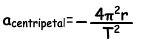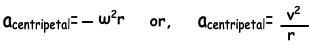Tangential Velocity;

V=2πr/time where r is the radius of the motion path and T is the period of the  motion

AngularVelocity;

ω=2π/T=2πf where T is the period of the  motion and f is the frequency

Angular Acceleration (Centripetal Acceleration);orwhere ω is the angular velovity, r is the radius and v is the tangential velocity

Centripetal Force;

Fc=-m4π²r/T² or Fc=mv²/r

Where, T is the the period, V is the tangential velocity and m is the mass of the object

Torque;

Τ=Applied Force.Distance.sinΘ

Τ=F.d.sinΘ

Rotational Motion Exams and Solutions

Related